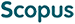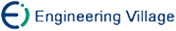杨 磊 宋 涛. 基于数组的桶排序算法[J]. 计算机研究与发展, 2007, 44(2): 341-347.
 引用本文: 杨 磊 宋 涛. 基于数组的桶排序算法[J]. 计算机研究与发展, 2007, 44(2): 341-347.Yang Lei and Song Tao. The Array-Based Bucket Sort Algorithm[J]. Journal of Computer Research and Development, 2007, 44(2): 341-347.
 Citation: Yang Lei and Song Tao. The Array-Based Bucket Sort Algorithm[J]. Journal of Computer Research and Development, 2007, 44(2): 341-347.## The Array-Based Bucket Sort Algorithm

• 摘要: 经典桶排序算法以链表形式实现“桶”，处理均匀数据效率很高，是O(N)算法.但对极不均匀数据则退化成低效的O(N\+2)插入排序.讨论了记录携带附加数据的计数排序算法，将“桶”实现为顺序数组，避免链表的动态内存分配直接提高算法效率，并允许快排等O(N log N)算法处理桶内数据.对均匀数据仍然保持O(N)时间复杂度，对极端不均匀数据则只退化为O(N log N)的原算法.对一般非均匀数据，证明数组桶排序算法总体性能高于经典算法.均匀数据实验表明，桶排序算法明显优于Linux下标准qsort系统调用，且数组桶排序算法效率更高.而在非均匀的正态数据实验中数组桶算法性能下降明显小于经典桶排序，总体效率仍然优于qsort的直接应用.

Abstract: Classical bucket sort algorithm implements buckets as dynamic lists. It sorts uniform data efficiently within O(N) time but degrades to the inefficient O(N\+2) insertion sort when handling extremely-nonuniform data. By analyzing counting sort with extra data, a method is presented to implement the buckets as sequential arrays. The efficiency is improved directly by avoiding the complex operations of dynamic memory allocation. Furthermore, O(N log N) algorithms like quicksort may be employed to manage each bucket. The composed algorithm still sorts uniform data within O(N) time but simply degrades to the original O(N log N) algorithm in the worst case. In general case, it is proved that the composed bucket sort algorithm always achieves better performance. Experiments on uniform data show the superiorities of the bucket sort algorithms, and that the array-based bucket sort algorithm beats the classical algorithm. In experiments with Gaussian data, the non-uniformity influences the array-based algorithm much less than the classical algorithm where both outperform the quicksort./下载:  全尺寸图片 幻灯片
• 分享
• 用微信扫码二维码

分享至好友和朋友圈